cancel
Showing results for
Did you mean:

# PSoC 5, 3 & 1New Contributor II

## Non linear equation

Hey, guys

I'm developing a VFD and need to apply voltage proportional to frequency, i did some calculus and need to solve something like "-1.19=-2*x+sin(2*x)" .

Does PSoC/cortex M3 is able to solve x ? Maybe Newton-Rapshon method

How can I apply this?

Thanks

1 SolutionHonored Contributor II

## Re: Non linear equation

JoSi,

If limit the phase to 80-90% of the operational range, the task simplifies. Starting from the equation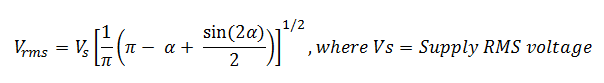and introducing normalized angle X=alpha/PI, and PFC = (V_rms/V_s)^2, we get:

PFC = 1 - x  +  sin(2*PI*x) / (2*PI);

Figure  1. Shows PFC factor as a function of X.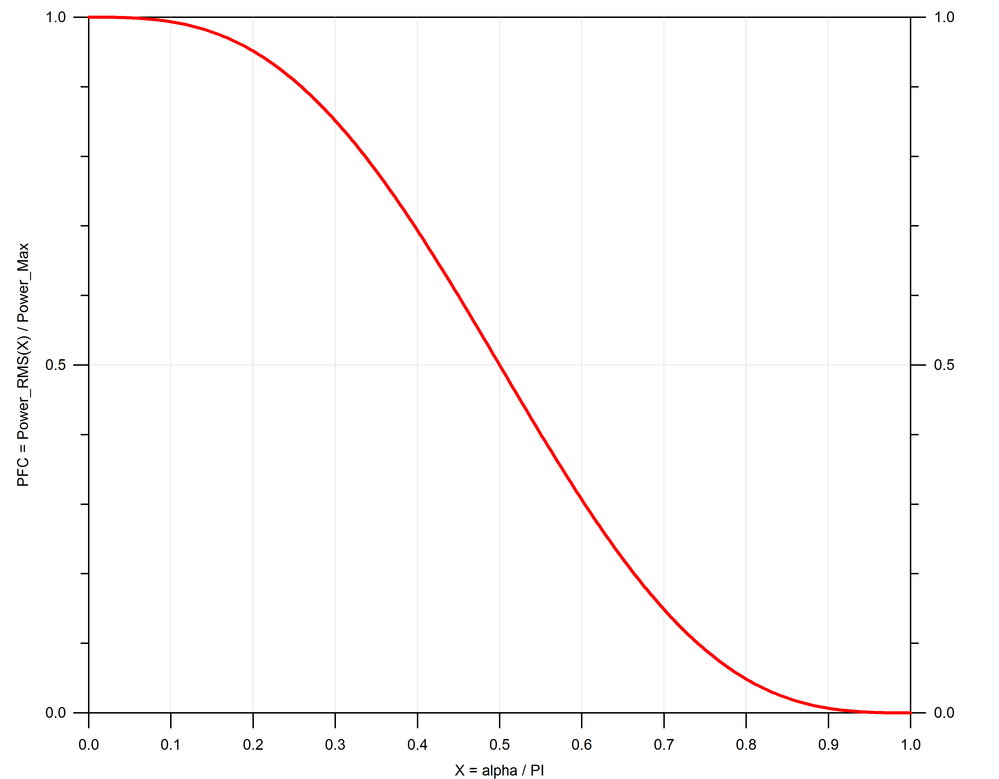Introducing Q2 = 0.5-PFC and X2 = X-0.5, the plot becomes centered against (0,0)

Fifure_2. Centered PFC plot.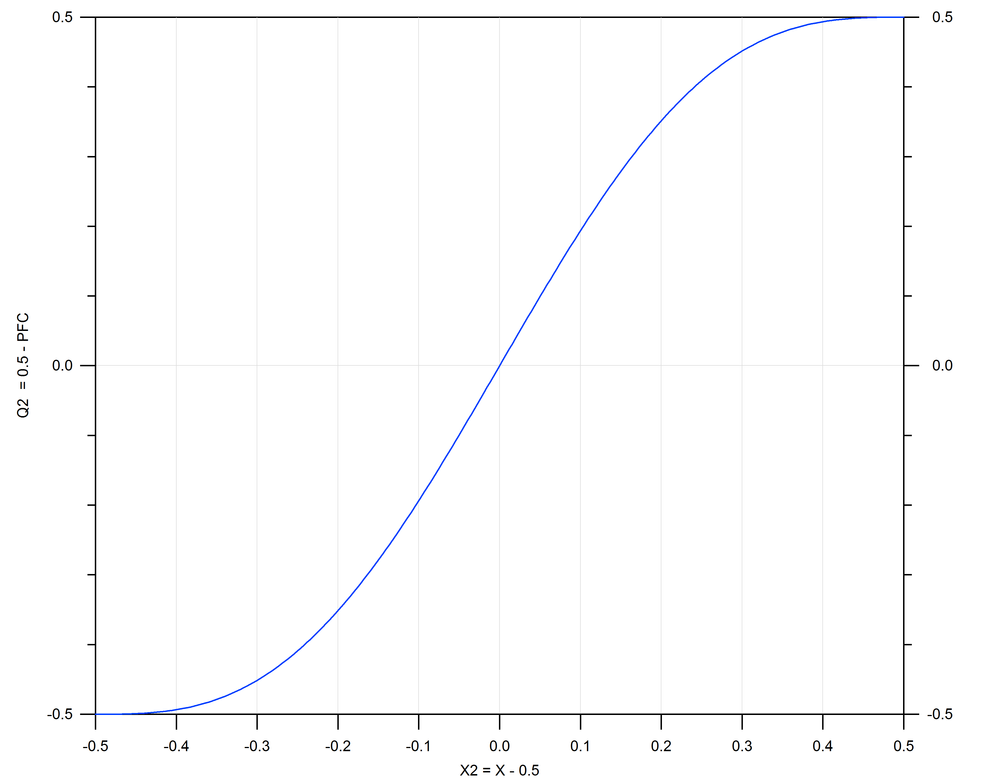Now exchange axis to get angle from PFC.

Figure 3. Shows angle (X2) vs PFC (Q2). It is very non-linear at the edges, but the central 80% portion of  Q2 fits well by poly4 function (red line). The curve span 0 to 257, centered at 128 (X=0).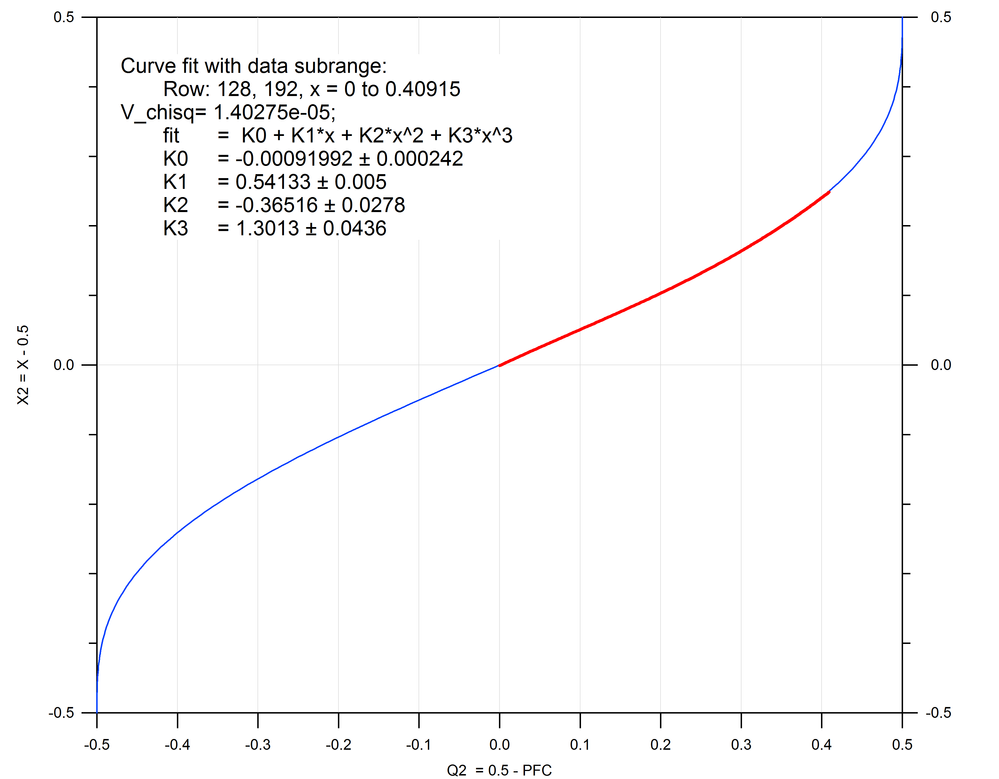Figure 4. The range can be expanded to 90% with loss of some accuracy (V_chisq ~1E-4, still good). Poly5 and Poly6 functions give better precision (chisq~1E-5) in same range, but do not let to expand the range - accuracy drops due to highly non-linear portion of the curve at the end of the range.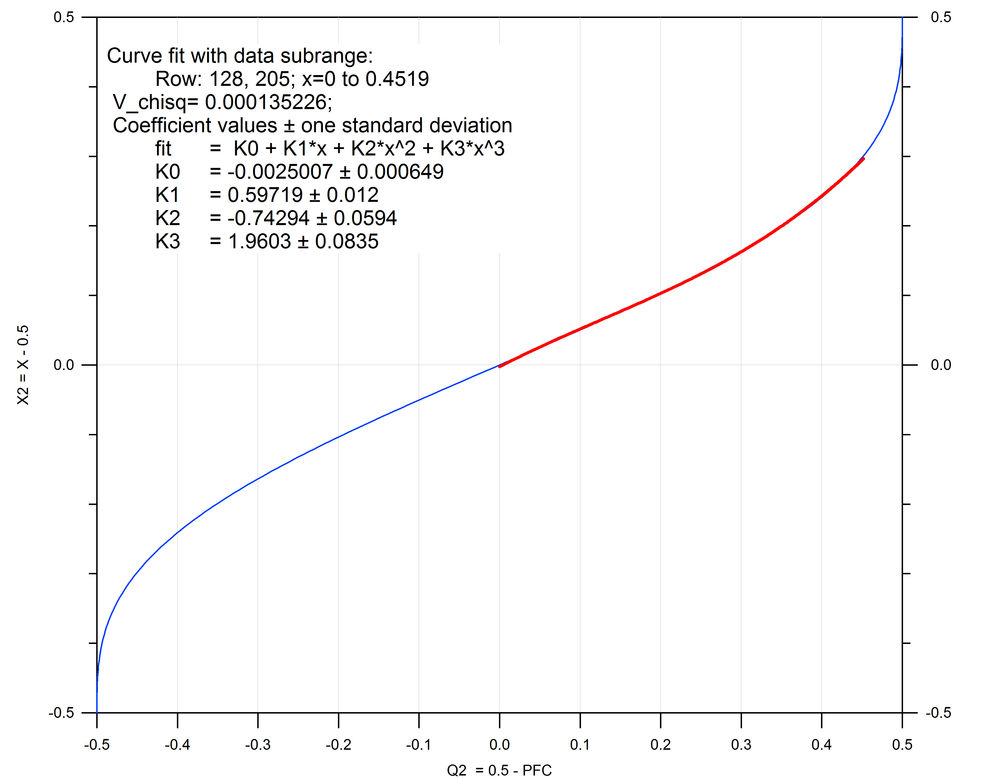The extremes of the range can be processed differently (maybe later).

So, if 80-90% is usable, then the recipe is simple:

1. Take desired PFC value and calculate Q2 = 0.5-PFC;

2. Calculate X2 using poly4 function.

3. Calculate angle X = X2+0.5.

Calculation should be relatively fast as it needs only ~6 multiplications, about 500 CPU clocks should suffice for the whole procedure. Further speedup can be achieved by making a lookup table for poly function. Let me know if higher order poly coefficients are needed for higher accuracy.

/odissey1

15 RepliesHonored Contributor II

## Re: Non linear equation

Equation

Y = 2x - Sin(2x);  // Y=1.19

Newton-Raphson iterations

X(n+1) = Xn - Y(Xn)/Y'(Xn)

Starting with approximated Xo~0.2, Yo=1.19

X(n+1) = Xn - (2Xn - Sin(2Xn)) / (2 - 2Cos(2Xn))

It should converge in 4-5 iterations, but is not fast due to Sin and Cos calculations.

Is it really necessary to use a non-linear equations in VFD control?

/odissey1

You may try simplified equation to speed-up calculus (unchecked equations):

X(n+1)=

= Xn - (2Xn - Sin(2Xn)) / (2 - 2Cos(2Xn))

= Xn - (1/4)(2Xn - Sin(2Xn)) / Sin^2(Xn)

Using Taylor series approximation

2x - Sin(2x) = (2x)^3/6 + ...

Sin^2(x)= (x - x^3/6+...)(x - x^3/6+...) = x^2 - 2(x^4)/6 + ...

= Xn - (1/4)((2Xn)^3/6) / Sin^2(Xn)

= Xn - (1/4)((2Xn)^3/6) / (x^2 - 2(x^4)/6)

= Xn - ((Xn^3)/3) / (Xn^2 - (Xn^4)/3)

= Xn - Xn / (3 - Xn^2)

Final

X(n+1)  = Xn - Xn / (3 - Xn^2)New Contributor II

## Re: Non linear equation

My idea is to apply a voltage proportional to frequency, so if my Vs=220V@60Hz and I want 30Hz in my load, I should apply 110V in Vrms to calculate alpha and fire a triac.

If there's a simple way to do this, please tell me.Honored Contributor II

## Re: Non linear equation

JoSi,

Calculations seem to be easy, just set a while loop until X(n+1)-X(n) < small_delta.

This is feed-forward type of control, it is hard to predict how well it will perform in real application. In some cases, the feed-forward control is supplemented with PID feedback loop to account for small inaccuracies. Basically, first calculate new setpoint and apply it as initial value, then let PID to fine-tune.

Instead, I would rather implement simple PI loop with input parameter as motor frequency and setpoint at 30Hz. Though PID-type control intended for linear systems, it might work OK if area of tuning is small (e.g. centered at 30Hz)

Check previous discussion on motor control here:

https://community.cypress.com/message/194446#194446

and here:

/odissey1Honored Contributor II

## Re: Non linear equation

JoSi,

If limit the phase to 80-90% of the operational range, the task simplifies. Starting from the equationand introducing normalized angle X=alpha/PI, and PFC = (V_rms/V_s)^2, we get:

PFC = 1 - x  +  sin(2*PI*x) / (2*PI);

Figure  1. Shows PFC factor as a function of X.Introducing Q2 = 0.5-PFC and X2 = X-0.5, the plot becomes centered against (0,0)

Fifure_2. Centered PFC plot.Now exchange axis to get angle from PFC.

Figure 3. Shows angle (X2) vs PFC (Q2). It is very non-linear at the edges, but the central 80% portion of  Q2 fits well by poly4 function (red line). The curve span 0 to 257, centered at 128 (X=0).Figure 4. The range can be expanded to 90% with loss of some accuracy (V_chisq ~1E-4, still good). Poly5 and Poly6 functions give better precision (chisq~1E-5) in same range, but do not let to expand the range - accuracy drops due to highly non-linear portion of the curve at the end of the range.The extremes of the range can be processed differently (maybe later).

So, if 80-90% is usable, then the recipe is simple:

1. Take desired PFC value and calculate Q2 = 0.5-PFC;

2. Calculate X2 using poly4 function.

3. Calculate angle X = X2+0.5.

Calculation should be relatively fast as it needs only ~6 multiplications, about 500 CPU clocks should suffice for the whole procedure. Further speedup can be achieved by making a lookup table for poly function. Let me know if higher order poly coefficients are needed for higher accuracy.

/odissey1New Contributor II

## Re: Non linear equation

Thanks one more time, BoTa_264741​, you are the man.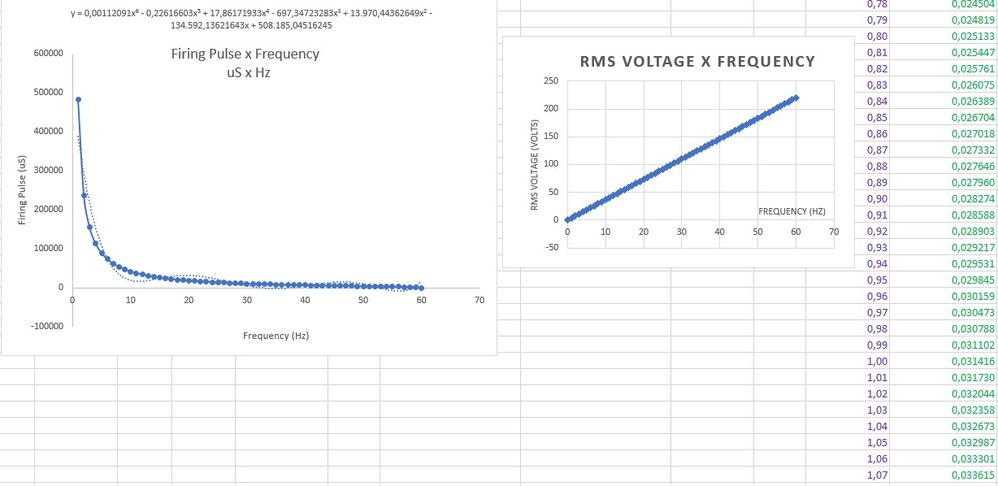dissey

I've done a lookup table in excel varying alpha (0 to pi) from 0% up to 100% in increments of 0.01 (gives me 10k values), then used the VLOOKUP function to complete another table to interpolate alpha in 60 values (0 to 60Hz), then used 6th order interpolation polynomial and get a function. I think this gonna workHonored Contributor II

## Re: Non linear equation

JoSi,

I am finally used poly20 to fit whole range to make a lookup table. Good to know that you already passed this point. I see some oscillations on first graph, where did they come from?

I am curious if this equation  works at all, since it was derived  for the resistive load, not active inductive load like a motor. Please let us know how well this approach worked with a motor.

odissey1New Contributor II

## Re: Non linear equation

BoTa_264741​, dotted line is from 6th order polynomial interpolation 'fitting' the curve

One more question:

The signals PWM_0 and PWM_180 are going to a H bridge and it's working like a charm, I'm able to vary the frequency output of the WaveDAC_Sine through the DDS32.

If I could change the WaveDAC_Sine amplitude (from 1.020 to 0.2, i.e) my voltage at H bridge will change too, right?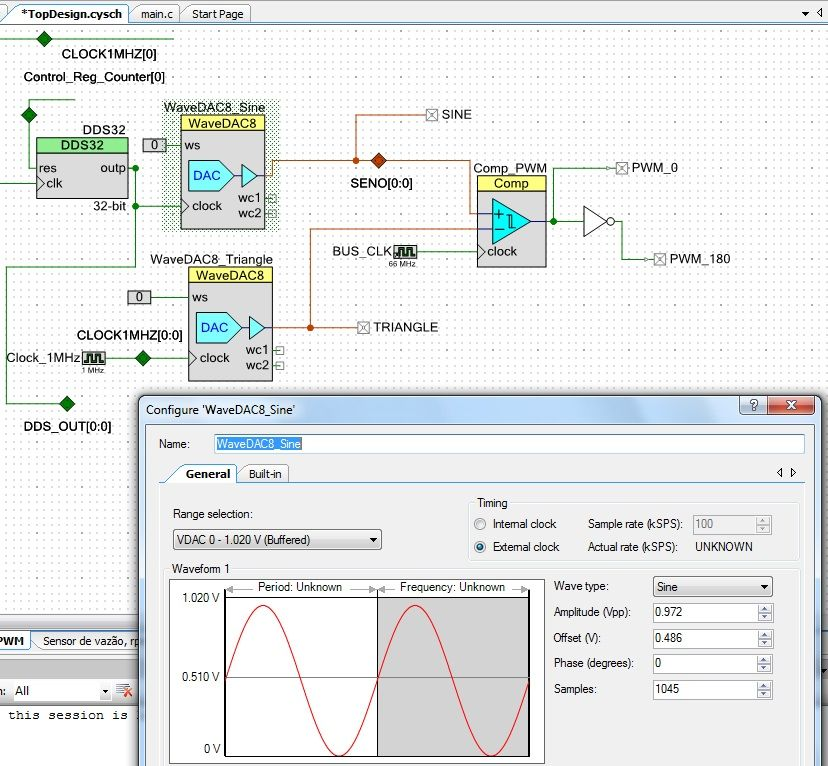Honored Contributor II

## Re: Non linear equation

JoSi,

>If I could change the WaveDAC_Sine amplitude (from 1.020 to 0.2, i.e) my voltage at H bridge will change too, right?

-Yes. This is "traditional" way of bridge control using a triangle wave and Comparator. You can find a demo project showing Sine wave amplitude control here:

Audio signal generator: strange noise with headphones, no noise when connected to PC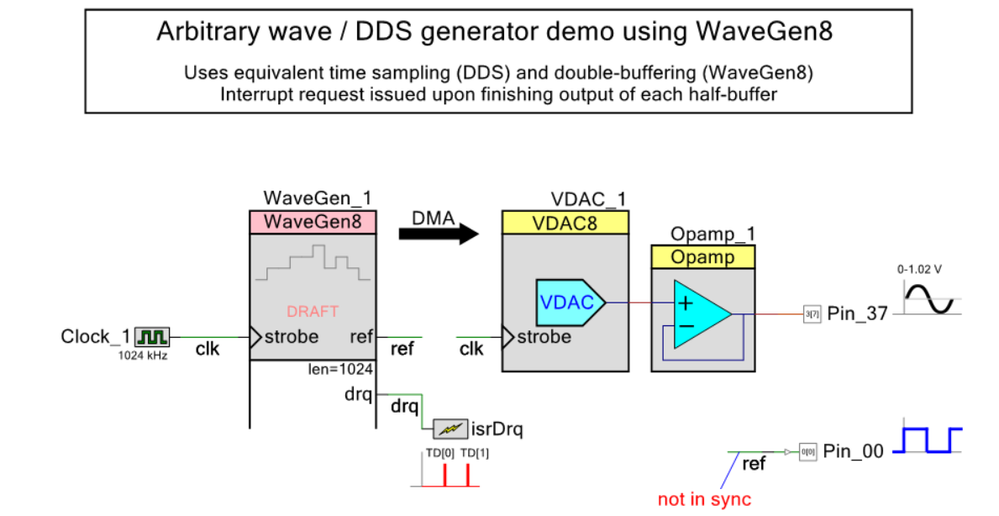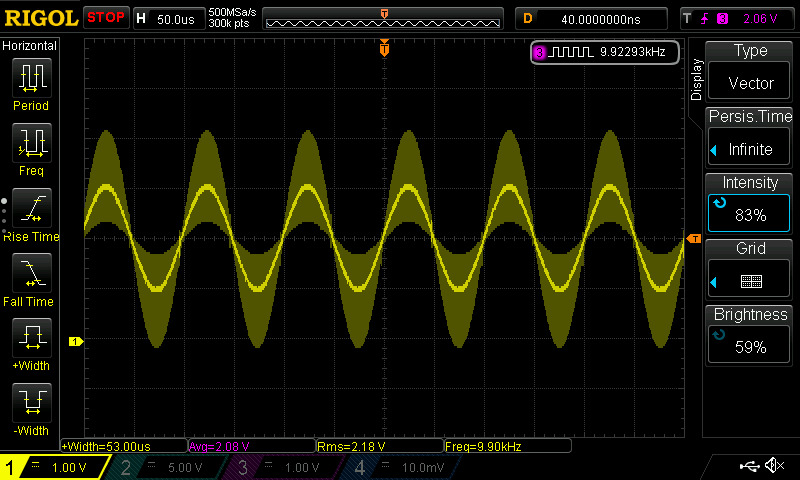It uses a custom component WaveGen to feed VDAC with data from RAM buffer using DMA. In essence, it is same as WaveDAC with less overhead.

But...

The output to the H-Bridge is a digital PWM waveform. It quite wasteful to produce simple PWM output using two analog generators.

/odissey1New Contributor II

## Re: Non linear equation

After my H bridge there's an LC filter (fc=800Hz)

"The output to the H-Bridge is a digital PWM waveform. It quite wasteful to produce simple PWM output using two analog generators."

So, what's the best way to produce SPWM without using two analog generators?Honored Contributor II

## Re: Non linear equation

JoSi,

Attached is example of producing PWM output to H-bridge by directly feeding 60Hz Sine wave from the RAM buffer to the PWM compare1. It uses a custom component (WaveGen), which is nothing but a simple DMA configurator. Attached project has two pages, one is configured for 256 sine points/period and another one is 32 sine points/period (this one is only to clarify signals on the scope). Please enable only one page at a time.

Project uses a cheap rotary encoder to update sine amplitude, phase and frequency. You can disable this functionality and use some fixed values. The WaveGen component is included into the project. Custom libraries (DDS32, QuadDecoder_SW and PSoC Annotation Library) can be downloaded here:

Re: DDS24: 24-bit DDS arbitrary frequency generator component

Re: Quad Decoder with Button Switch component for rotary shaft encoders

PSoC Annotation Library v1.0

/odissey1

P.S. Attached is some Phase Controlled Converters educational materials. It has all bunch of equations for PFC control of Resistive, Inductive and Capacitive loads, which may be useful.

Figure 1. Project schematics. Markers (Yellow, Cyan and Fuchsia squares) indicate scope trace color. Notice phase outputs from the PWM (ph1, ph2), which can drive H-bridge directly with deadtime insertion.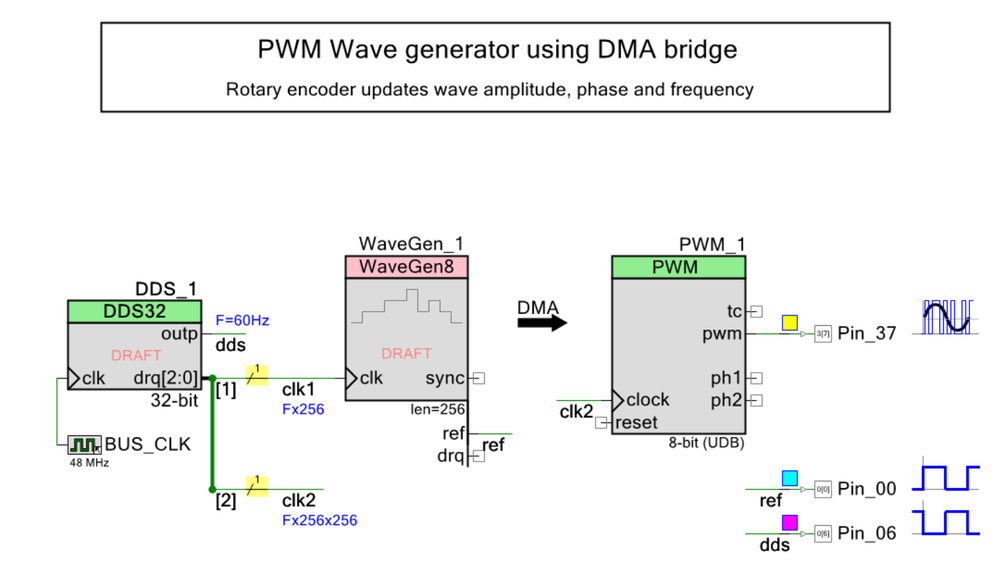Figure 2. Yellow trace - PWM output to the bridge (effective sine wave amplitude +127 with 32 points/period). Cyan trace - WaveGen reference output (60Hz).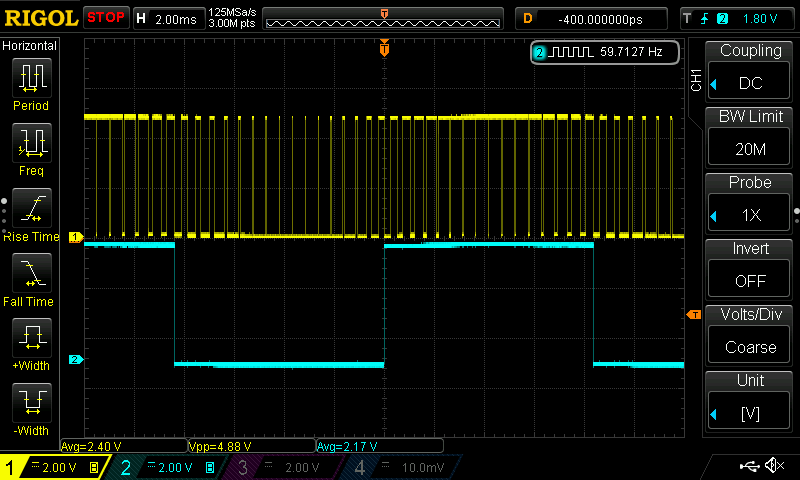Figure 3. KIT-059 pins output (uses PSoC Annotation Library)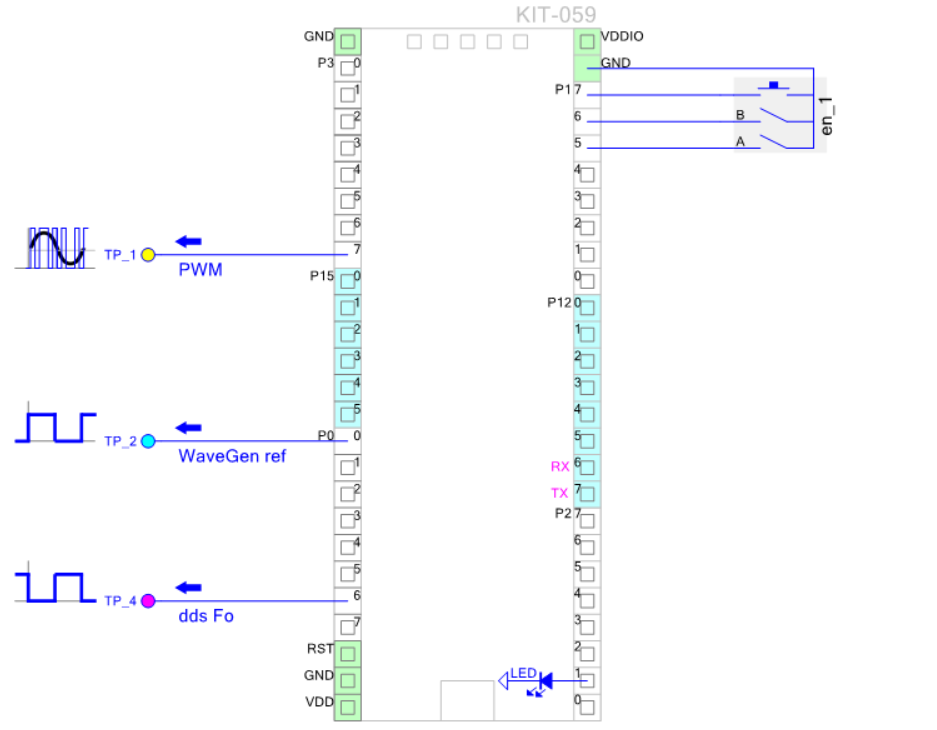Honored Contributor II

## Re: Non linear equation

JoSi,

I figured out that PWM output waveform may depend on H-bridge driver type. Can you please post the part number for the H-bridge driver used? Also, one should be careful not to exceed H-bridge specs limitations, typically PWM max frequency and turn-ON, turn-OFF time.

/odissey1New Contributor II

## Re: Non linear equation

My setup is:

4x MOSFET IRF840

Driver: IR2110Honored Contributor II

## Re: Non linear equation

JoSi,

There is Wiki article re: VFD AC motor control: Variable-frequency drive - Wikipedia

And the PWM output from the project above (yellow trace) looks similar to the Wiki page (pink line).But...

In the project posted above I used 8-bit sine wave centered at 128.

WaveGen_1_CreateSineWave_C(ampl, phase);  // Sine wave centered at 128, A(t)=128 + ampl * sine(2*PI* t/T); ampl=[-127, +127]

I think that to correctly vary the torque using full H-bridge, the sine wave should be bottom-aligned instead, like this:

WaveGen_1_CreateSineWave_B(ampl, phase);  // bottom-aligned Sine wave, A(t)= 0.5 * ampl * (1 + sine(2*PI* t/T)); ampl=[0, 255]

/odissey1Honored Contributor II

## Re: Non linear equation

JoSi,

I found a useful blog about H-bridge controls, which goes into fine details of the subject, discussing various modes of the operation

H-Bridge Secrets | Modular Circuits

I am also attaching one of the articles discussed there.

/odissey1New Contributor II

## Re: Non linear equation